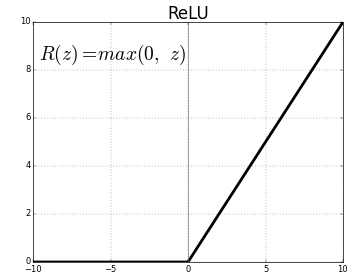# Why is ReLU used as an activation function?

Activation functions are used to introduce non-linearities in the linear output of the type w * x + b in a neural network.

Which I am able to understand intuitively for the activation functions like sigmoid.

I understand the advantages of ReLU, which is avoiding dead neurons during backpropagation. However, I am not able to understand why is ReLU used as an activation function if its output is linear?

Doesn't the whole point of being the activation function get defeated if it won't introduce non-linearity?

• This post is 2 years old but I'd like to mention that ReLU does not avoid dead neurons. "Dead" neurons occur due the 0 gradient of the ReLU function when the input is less than 0. May 25 '20 at 18:05
• Right, there's a modified version of ReLU which helps to avoid "dead" neurons. It's called LeakyReLU and it has a slightly sloped activation for negative numbers. Dec 18 '20 at 18:08

In mathematics (linear algebra) a function is considered linear whenever a function$$f: A \rightarrow B$$ if for every $$x$$ and $$y$$ in the domain $$A$$ has the following property: $$f(x) + f(y) = f(x+y)$$. By definition the ReLU is $$max(0,x)$$. Therefore, if we split the domain from $$(-\infty, 0]$$ or $$[0, \infty)$$ then the function is linear. However, it's easy to see that $$f(-1) + f(1) \neq f(0)$$. Hence by definition ReLU is not linear.

Nevertheless, ReLU is so close to linear that this often confuses people and wonder how can it be used as a universal approximator. In my experience, the best way to think about them is like Riemann sums. You can approximate any continuous functions with lots of little rectangles. ReLU activations can produced lots of little rectangles. In fact, in practice, ReLU can make rather complicated shapes and approximate many complicated domains.

I also feel like clarifying another point. As pointed out by a previous answer, neurons do not die in Sigmoid, but rather vanish. The reason for this is because at maximum the derivative of the sigmoid function is .25. Hence, after so many layers you end up multiplying these gradients and the product of very small numbers less than 1 tend to go to zero very quickly.

Hence if you're building a deep learning network with a lot of layers, your sigmoid functions will essentially stagnant rather quickly and become more or less useless.

The key take away is the vanishing comes from multiplying the gradients not the gradients themselves.

• f(x) = 2*x + 3 is a linear function but does it satisfies the propetry f(x)+f(y) = f(x+y)? Mar 21 '20 at 10:19
• @flamelite 2x + 3 is actually NOT a linear function. It is an affine function. In high school algebra classes it would probably be called linear because its graph is a line, but it isn't. Apr 16 '20 at 18:09

I understand the advantages of ReLU, which is avoiding dead neurons during backpropagation.

This is not completely true. The neurons are not dead. If you use sigmoid-like activations, after some iterations the value of gradients saturate for most the neurons. The value of gradient will be so small and the process of learning happens so slowly. This is vanishing and exploding gradients that has been in sigmoid-like activation functions. Conversely, the dead neurons may happen if you use ReLU non-linarity, which is called dying ReLU.

I am not able to understand why is ReLU used as an activation function if its output is linear

Definitely it is not linear. As a simple definition, linear function is a function which has same derivative for the inputs in its domain.

The linear function is popular in economics. It is attractive because it is simple and easy to handle mathematically. It has many important applications. Linear functions are those whose graph is a straight line. A linear function has the following property:

$$f(ax + by) = af(x) + bf(y)$$

ReLU is not linear. The simple answer is that ReLU's output is not a straight line, it bends at the x-axis. The more interesting point is what’s the consequence of this non-linearity. In simple terms, linear functions allow you to dissect the feature plane using a straight line. But with the non-linearity of ReLUs, you can build arbitrary shaped curves on the feature plane.

ReLU may have a disadvantage which is its expected value. There is no limitation for the output of the Relu and its expected value is not zero. Tanh was more popular than sigmoid because its expected value is equal to zero and learning in deeper layers occurs more rapidly. Although ReLU does not have this advantage batch normalization solves this problem.

• f(x) = a + bx is not a linear function unless a is 0. Apr 16 '20 at 18:13
• @BradyGilg Nice catch! Apr 16 '20 at 18:32

The main reason to use an Activation Function in NN is to introduce Non-Linearity. And ReLU does a great job in introducing the same.Three reasons I choose ReLU as an Activation Function

• First it's Non-Linear( although it's acts like a linear function for x > 0)
• ReLU is cheap to compute. Since it's simple math, model takes less time to run
• ReLU induces sparsity by setting a min value of 0
• Can you explain sparsity in this context?
– Shiv
Sep 16 '20 at 5:14